# What is an Electromagnet (Explained Simply)

## Introduction:

Electromagnetism is one of the important characteristics of a current carrying conductor. As stated by the Hans Christian Oersted in his theory “Magnetic Effect of Current Carrying Conductor,” whenever a current flows in a conductor, it produces a magnetic field around the conductor

As soon as the current is removed the magnetic filed created in the conductor also disappears. Thus it is called a temporary magnet or simple electromagnet.

## Simple Electromagnet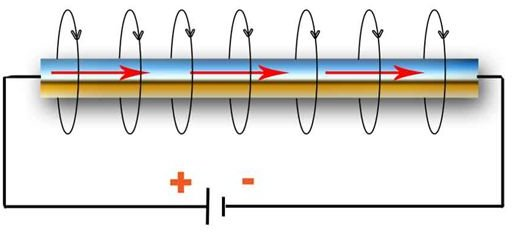## Solenoid

To increase the magnetic intensity of a simple electromagnet, we can include a long closely wounded helical coil. This creates a solenoid. As the electromagnet is a temporary magnet, when the current is passed through the solenoid coils it behaves like a magnet, and it de-magnetizes as soon the current is removed.

The magnetic field in the solenoid is the vector sum of the magnetic fields due to current through individual turns of the solenoid. The magnetic fields associated with each single turn almost concentric circles and hence tend to cancel between the turns. At the interior mid-point, the field is strong and along the axis of the solenoid.

In simple words for a point P, the field due to the upper part of the solenoid turns tends to cancel the field due to the lower part of the solenoid turns, acting in opposite directions. Hence the field outside the solenoid is nearly zero.

Further by introducing the core of ferromagnetic material such as iron, we can increase the magnetic field produced by the solenoid. Due to high magnetic permeability of the iron or ferromagnetic materials, the magnetic field produced by the solenoid will be further increased by more than a thousand times the field produced.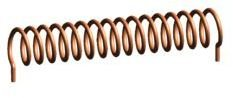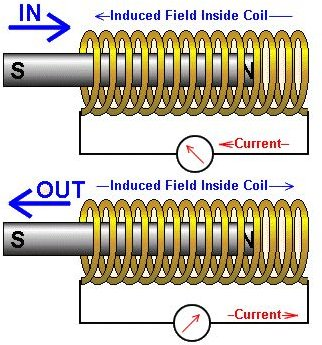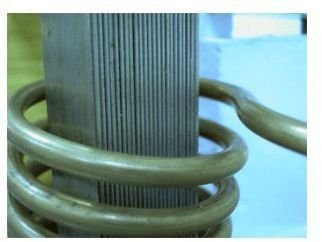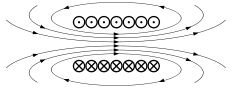## Principles Used In Solenoids

Right Hand Palm Rule

The direction of magnetic field due to circular closed loop in solenoid is given by the Right Hand Palm Rule:

The coil is held in the right hand so that the fingers point in the direction of current in the windings. The extended thumb points in the direction of the magnetic field.

End Rule

The magnetic polarity of the current carrying solenoid is given by the End Rule.

• When looked from one end, if the current through the solenoid is along clockwise direction the nearer end corresponds to South Pole and the other end is North Pole.
• When looked from one end, if the current through the solenoid is along anti-Clock wise direction, the nearer end corresponds to North Pole and other end is South Pole.

## Right Hand Palm Rule and End Rule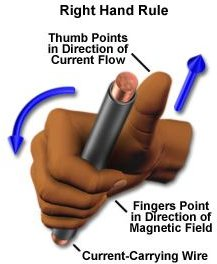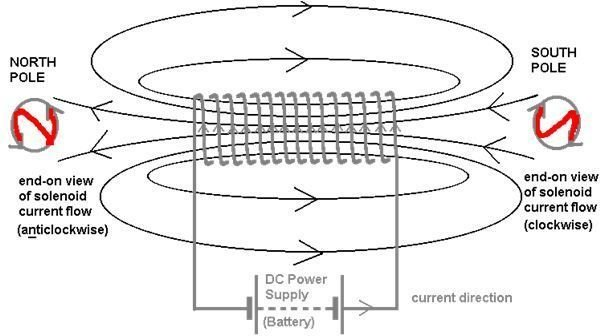## Toroid

Toroid is also one type of electromagnet commonly used in toroidal inductors and transformers. Normally, these toroid types of electromagnet have higher inductance than the solenoid type of electromagnet for the same number of turns.

Toroid is a hollow circular ring on which a large number of turns are closely wounded. It can be considered as a long solenoid bent in the form of a closed ring.

In this case the magnetic field in different positions of the toroid is:

• Magnetic induction at any point inside the toroid (that is along the axis or in the iron core part) will be maximum.
• Magnetic induction at any point in open space inside the toroid will be zero.
• Magnetic induction at any point in open space outside the toroid will be zero.

## Toroid: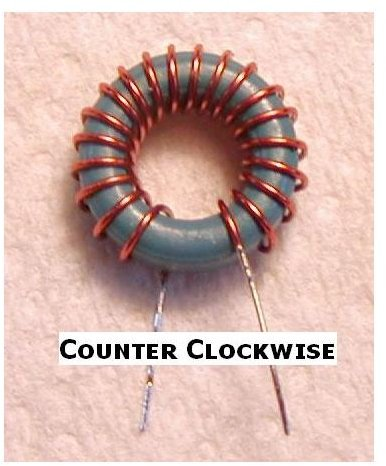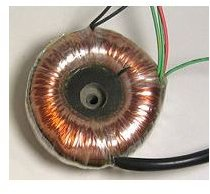Image Credit:

https://kenolab.com/projects/Magnetic%20Transmitter/index.html

https://www.kitsandparts.com/howtowindtoroidswithoutpain.php

https://en.wikipedia.org/wiki/Toroidal_inductors_and_transformers

https://en.wikipedia.org/wiki/Solenoid

https://www.magnet.fsu.edu/education/tutorials/java/handrules/index.html

https://www.cyberphysics.co.uk/topics/magnetsm/Electromagntism.htm

https://www.societyofrobots.com/actuators_solenoids.shtml